# Intuition of SpanBert

Prerequisite: BERT Model

SpanBERT vs BERT

SpanBERT is an improvement on the BERT model providing improved prediction of spans of text. Unlike BERT, we perform the following steps here i) mask random contiguous spans, rather than random individual tokens.
ii) training the model based on tokens at the start and end of the boundary of span (known as Span Boundary Objective) to predict the entire marked spans.

It differs from BERT model in its masking scheme as BERT used to randomly mask tokens in a sequence but here in SpanBERT we mask random contiguous spans of text.

Another difference is that of the training objective. BERT was trained on two objectives (2 loss functions) :

• Next Sequence Prediction (NSP) — Predicting if 2 sequences of texts followed each other.

But in SpanBERT, the only thing the model is trained on is the Span Boundary Objective which later contributes to the loss function.

SpanBERT: Implementation

To implement SpanBERT, we build a replica of the BERT model but do certain changes in it so that it can perform better than the original BERT model. It is observed that the BERT model performs much better when only trained on ‘Masked Language Modelling’ alone rather than with ‘Next Sequence Prediction’. Hence, we disregard NSP and tuned the model on Single Sequence baseline while building the replica of BERT model, thereby improving its prediction accuracy.

SpanBERT: Intuition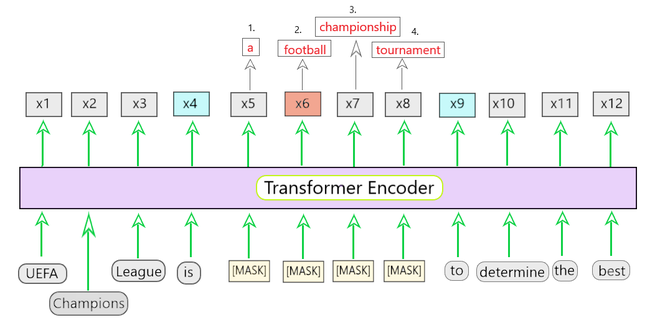Fig 1 : Training SpanBERT

Fig 1 shows the training of the SpanBERT model. In the given sentence, the span of words ‘a football championship tournament‘ is masked. The Span Boundary Objective is defined by the x4 and x9 highlighted in blue. This is used to predict each token in the masked span.

Here in Fig 1, a sequence of words ‘a football championship tournament‘ is created and the whole sequence is passed through the encoder block and get the prediction of the masked tokens as output. (x5 to x8

For example, if we were to predict for the token x6 (i.e. football), below is the equivalent loss (as shown in Eqn(1)) that we would get.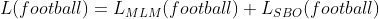Eqn-(1)

This loss is the summation of losses given by MLM and SBO losses.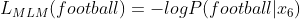Eqn-(2)

Now, the MLM loss is the same as ‘-ve log of likelihood’ or in simpler terms what is the chances of x6 being football.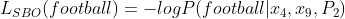Eqn-(3)

```Then, the SBO loss is depends on three parameters.

x4 - the start of the span boundary
x6 - the end of the span boundary
P2 - the position of x6 (football) from the starting point (x4)

So given these three parameters, we see how good the model is at predicting the token 'football'.
```

Using the above two loss functions, the BERT model is fine-tuned and is called SpanBERT.

Span Boundary Objective:

Here, we get the output as a vector encoding the tokens in the sequence represented as (x1, ….., xn). The masked span of tokens is represented by (xs, …., xe), where xs denotes start and xe denotes the end of the masked span of tokens. SBO function is represented as: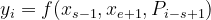Eqn-(4)

```where P1, P2, ... are relative positions w.r.t the left boundary token xs-1.
```

The SBO function ‘f’ is a 2 layer feed-forward network with GeLU activation. This 2 layer network is represented as: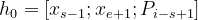Eqn-(5)

```where,

h0 = first hidden representation
xs-1 = starting boundary word
xe+1 = ending boundary word
Pi-s+1 = positional embedding of the word
```

We pass h0 to first hidden layer with weight W1.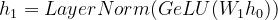Eqn-(6)

```where,

GeLU (Gaussian Error Linear Units) = non-linear activation function
h1 = second hidden representation
W1 = weight of first hidden layer
LayerNorm = a normalization technique used to prevent interactions within the batches
```

Now, we pass this through another with weight W2 layer to get the output yi.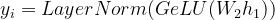Eqn-(7)

```where,
yi = vector representation for all the toxens xi
W2 = wight of second hidden layer
```

To generalize, SpanBERT equivalent loss of a particular token in a span of words is calculated by: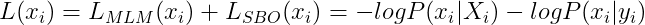Eqn-(8)

```where,
Xi = final representation of tokens
xi = original sequence of tokens
yi = output obtained by passing xi through 2-layer feed forward network.
```

This was a basic intuition and understanding of the SpanBERT model and how it predicts a span of words instead of the individual token, making it more powerful than the BERT Model. For any doubts/queries, comment below.

My Personal Notes arrow_drop_upCheck out this Author's contributed articles.

If you like GeeksforGeeks and would like to contribute, you can also write an article using contribute.geeksforgeeks.org or mail your article to contribute@geeksforgeeks.org. See your article appearing on the GeeksforGeeks main page and help other Geeks.

Please Improve this article if you find anything incorrect by clicking on the "Improve Article" button below.

Article Tags :
Practice Tags :

Be the First to upvote.

Please write to us at contribute@geeksforgeeks.org to report any issue with the above content.